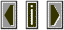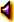Beat FrequenciesBeat frequencies, or difference tones, result when two frequencies which are very close in frequency occur simultaneously.The two tones will pulse at a frequency which is the difference of the two original frequencies. Thus if a tone of 30 Hertz is sounded simultaneously with another tone of 34 Hertz, "beating" will occur at a frequency of 4 Hertz (34-30=4).

 In the following  sound  example there are two tones starting together at 1000 Hz. One of the two tones then shifts pitch down and up to reveal beating between the two tones.
Sound Example:beating example